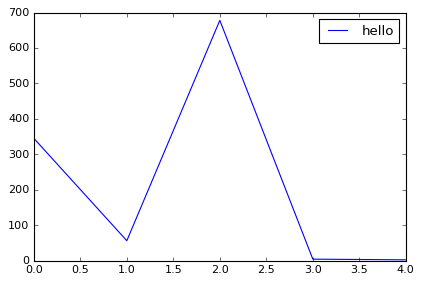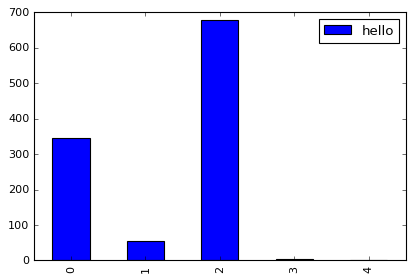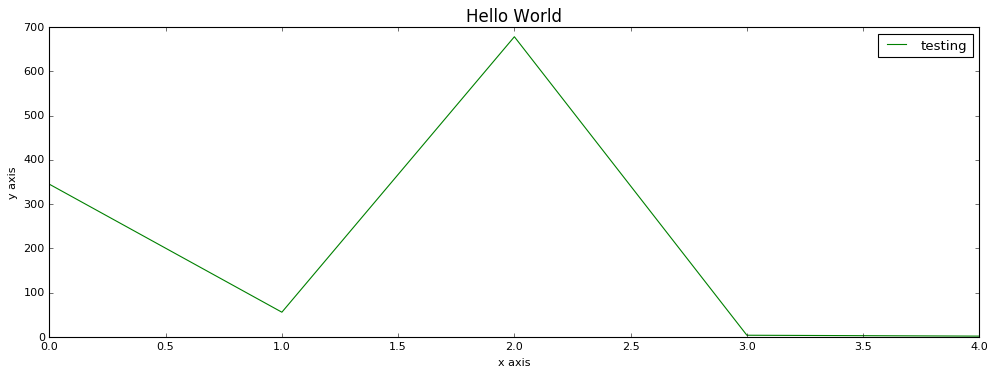Hedaro Blog

# Pandas Plot - How to Create a Basic Pandas Visualization

In this lesson we will learn how to create a basic pandas plot. Discover why MatPlotLib is Python's default charting library and how it is used to create Pandas visualizations.

Pandas is built on top of Numpy and MatPlotLib. It uses MatPlotLib for most of its charting capabilities.

Tip: If you ever have a plotting question and are not finding an answer. Try searching for it using the MatPlotLib keyword instead of Pandas.

Let's start by importing Pandas. Note that we do not have to import MatPlotLib as it is already part of Pandas. But in order to get the version of MatPlotLib installed on my machine, I need it available.

What about that last import %matplotlib inline?
This line of code allows your charts and plots appear in the Notebook. Without this piece of code, you will not see any Padnas plots.

If you want to configure the Notebook to always import certain libraries, you can. You can google around for the specifics but I personally avoid any custom configurations.

In :
import pandas as pd
import matplotlib as mpl
import sys
%matplotlib inline


Here are the versions of the libraries I'm currently on.

In :
print('Python: ' + sys.version.split('|'))
print('Pandas: ' + pd.__version__)
print('MatPlotLib: ' + mpl.__version__)

Python: 3.5.1
Pandas: 0.23.4
MatPlotLib: 1.5.1


This tutorial is also available in video form. I try to go in more detail in the notebook but the video is worth watching.

In :
from IPython.display import YouTubeVideo

Out:

Let's steamroll straight into creating our dataframe. We are going to be creating a Pandas dataframe out of a Python dictionary. If you haven't seen my blog on creating Pandas dataframes, I encourage you to do that before moving on.

Here we have a one column dataframe with a few numeric rows. This is the data we will be plotting.

In :
df = pd.DataFrame({'hello':[345,56,678,4,2]})
df

Out:
hello
0 345
1 56
2 678
3 4
4 2

## Plotting in Pandas

Plotting in Pandas is actually very easy to get started with. The very basics are completely taken care of for you and you have to write very little code. It does get a bit tricky as you move past the basic plotting features of the library. This is where google is your friend.

By simply adding .plot(), you have yourself a Pandas visualization. We used to be able to resize the plot with the mouse but it seems that feature has been disabled.

In :
df.plot()

Out:matplotlib.axes._subplots.AxesSubplot at 0x91ca5c0 ???
Yes, I feel your pain. Why is this even here? Well, this matplotlib object will always show up and googling did not really give me a clear answer of the meaning of it. Do any of you have an idea?

Thr good news is that we can get rid of that pesky text by adding a semi colon at the end of our code cell like shown below.

In :
df.plot();## Selecting Chart Type

In the past we were able to create different types of charts by using the parameter called kind. But now Pandas makes it even easier. Let me illustrate the two.

By setting the kind parameter to bar, we get a bar chart.

In :
df.plot(kind='bar');You can now pass the chart types as methods to plot and this makes it much easier. I recommend you discontinue the use of the kind parameter going forward.

Tip: Type df.plot. and then press the Tab key on your keyboard. This will show you all of the chart options.

In :
df.plot.bar();When you are actually sifting through data and are concentrating, the default dot plot Pandas function works just fine. The only time you really want to style your plots is when you plan on presenting your work. This is where it becomes valuable to make things look pretty and readable to as many people as possible. Below we will go over a few examples to help you get there.

Oddly enough, setting your plots on avariable makes this process a breeze.

• We start by adjusting the figure size. You can use the figsize parameter and select the width and height of your visualizations.

• We can also pass into the plot function the color parameter and change the default line color of the plot.

• The set_title function allows you to title your charts. You can also change the font size to your liking.

• The set_xlabel function allows you to title the x axis. You can also use the fontsize parameter here.

• The set_ylabel function allows you to title the t axis. You can also use the fontsize parameter here.

• The legend function accepts a Python list and lets you customize the legend labels.

In :
p = df.plot(figsize=(15,5), color='green')

p.set_title('Hello World', fontsize=15)
p.set_xlabel('x axis')
p.set_ylabel('y axis')
p.legend(['testing']);And that is it for today. You should have enough horsepower to get started on your own plots. If your plots don't work on don't look quite right, keep on going. I was just using .plot() for years and I then picked up more plotting skills over time.

This tutorial was created by HEDARO